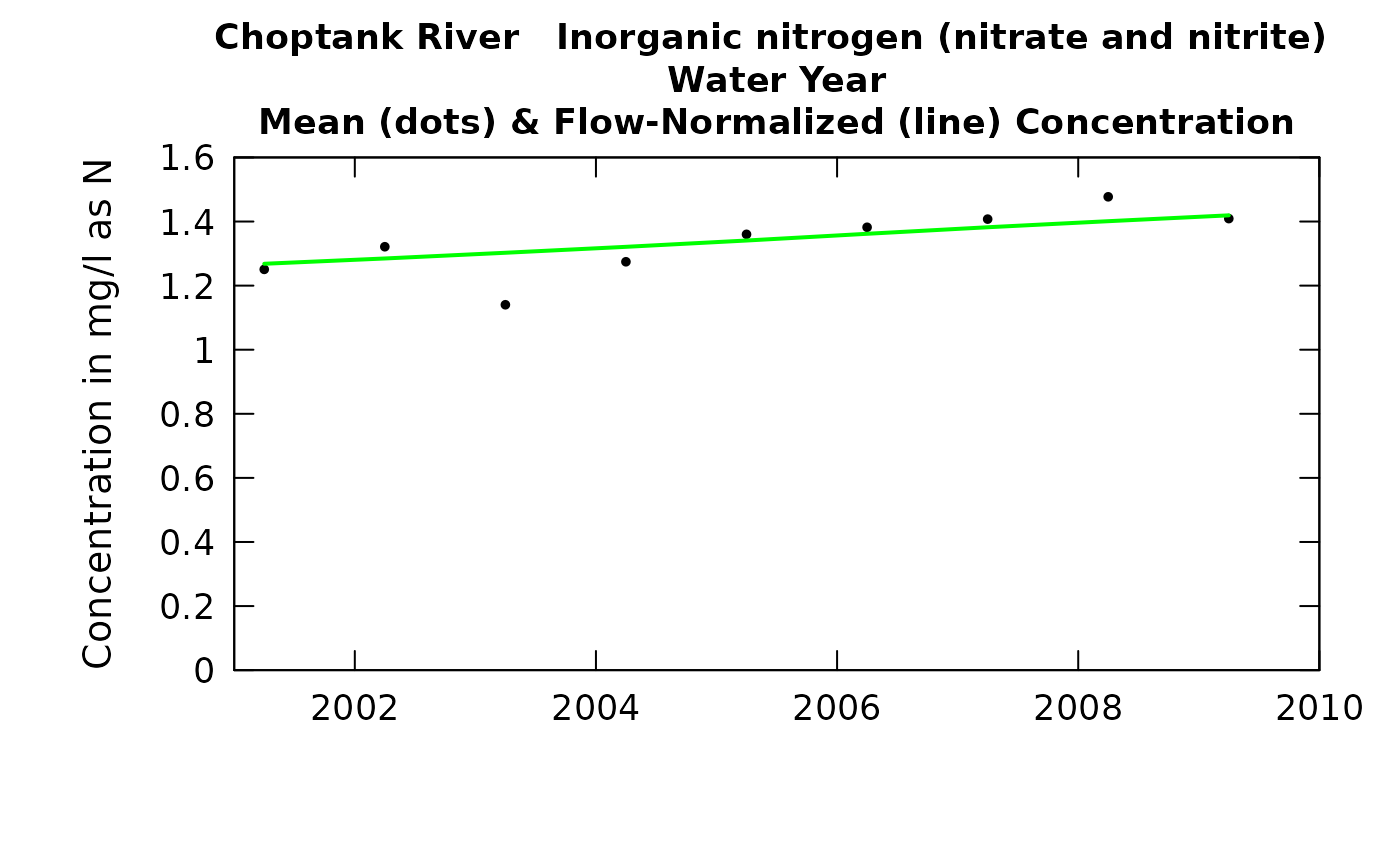Data come from named list (eList), which contains a Daily dataframe with the daily flow data, and an INFO dataframe with metadata.

The annual concentrations are "time-weighted" mean concentrations (as opposed to "flow-weighted"). The annual results reported are for a specified "period of analysis" which can be an entire water year, a calendar, a season or even an individual month. User specifies this period of analysis in the call to setupYears.

User can specify plotting of three possible series. All are in units of mg/L. Annual mean concentration WRTDS_K version of annual mean concentration (requires that WRTDSKalman has been run) Flow normalized mean concentration

Although there are a lot of optional arguments to this function, most are set to a logical default.

## Usage

plotConcHist(eList, yearStart = NA, yearEnd = NA, concMax = NA,
printTitle = TRUE, tinyPlot = FALSE, usgsStyle = FALSE,
plotFlowNorm = TRUE, plotAnnual = TRUE, plotGenConc = FALSE,
cex = 0.8, cex.axis = 1.1, cex.main = 1.1, lwd = 2, col = "black",
col.pred = "green", concLab = 1, col.gen = "red", customPar = FALSE,
...)

## Arguments

eList

named list with at least the Daily and INFO dataframes

yearStart

numeric is the calendar year containing the first estimated annual value to be plotted, default is NA (which allows it to be set automatically by the data)

yearEnd

numeric is the calendar year just after the last estimated annual value to be plotted, default is NA (which allows it to be set automatically by the data)

concMax

numeric. Maximum value of concentration to be plotted.

printTitle

logical variable if TRUE title is printed, if FALSE title is not printed (this is best for a multi-plot figure)

tinyPlot

logical variable, if TRUE plot is designed to be plotted small, as a part of a multipart figure, default is FALSE

usgsStyle

logical option to use USGS style guidelines. Setting this option to TRUE does NOT guarantee USGS compliance. It will only change automatically generated labels

plotFlowNorm

logical variable if TRUE flow normalized line is plotted, if FALSE not plotted

plotAnnual

logical variable if TRUE, annual concentration points from WRTDS output are plotted, if FALSE not plotted

plotGenConc

logical variable. If TRUE, annual concentration points from WRTDSKalman output are plotted, if FALSE not plotted

cex

numerical value giving the amount by which plotting symbols should be magnified

cex.axis

magnification to be used for axis annotation relative to the current setting of cex

cex.main

magnification to be used for main titles relative to the current setting of cex

lwd

number magnification of line width.

col

color of points on plot, see ?par 'Color Specification'

col.pred

color of flow normalized line on plot, see ?par 'Color Specification'

concLab

object of concUnit class, or numeric represented the short code, or character representing the descriptive name. By default, this argument sets concentration labels to use either Concentration or Conc (for tiny plots). Units are taken from the eList$INFO$param.units. To use any other words than "Concentration" see vignette(topic = "units", package = "EGRET").

col.gen

color of points for WRTDS_K output on plot, see ?par 'Color Specification'

customPar

logical defaults to FALSE. If TRUE, par() should be set by user before calling this function (for example, adjusting margins with par(mar=c(5,5,5,5))). If customPar FALSE, EGRET chooses the best margins depending on tinyPlot.

...

arbitrary graphical parameters that will be passed to genericEGRETDotPlot function (see ?par for options)

setupYears, genericEGRETDotPlot
yearStart <- 2001